# 仿射变换#

## 变换#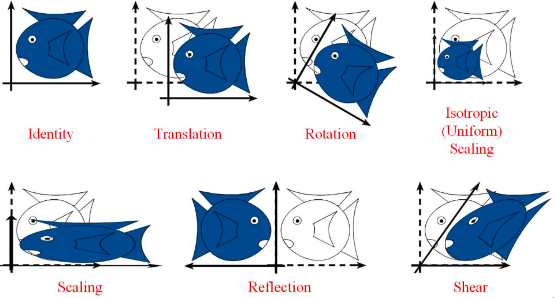• 平移，translation

• 旋转，rotation

• 缩放，scaling。如果每个坐标方向的缩放系数相同（即各向同性，isotropic），则为 uniform scaling

• 反射，reflection，关于某坐标轴对称。反射也可以看成是缩放的一个特例。

• 剪切变换，shear mapping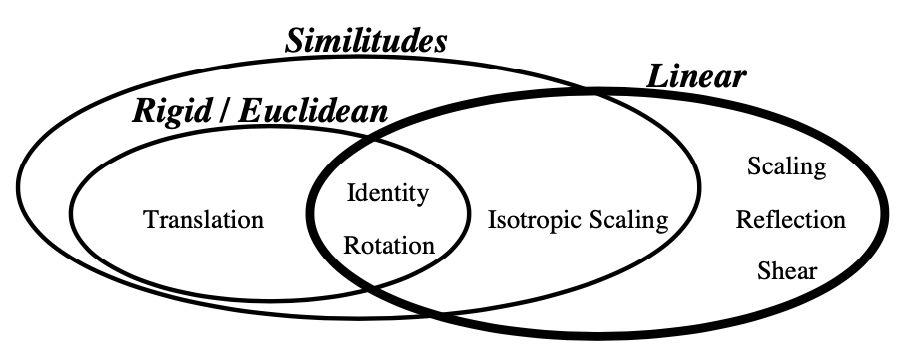• 旋转

• 反射

• 剪切

• 各向同性或者不同性的缩放

$\begin{split} \begin{split}&L(p+q)=L(p)+L(q)\\&L(\alpha p)=\alpha L(p)\end{split} \end{split}$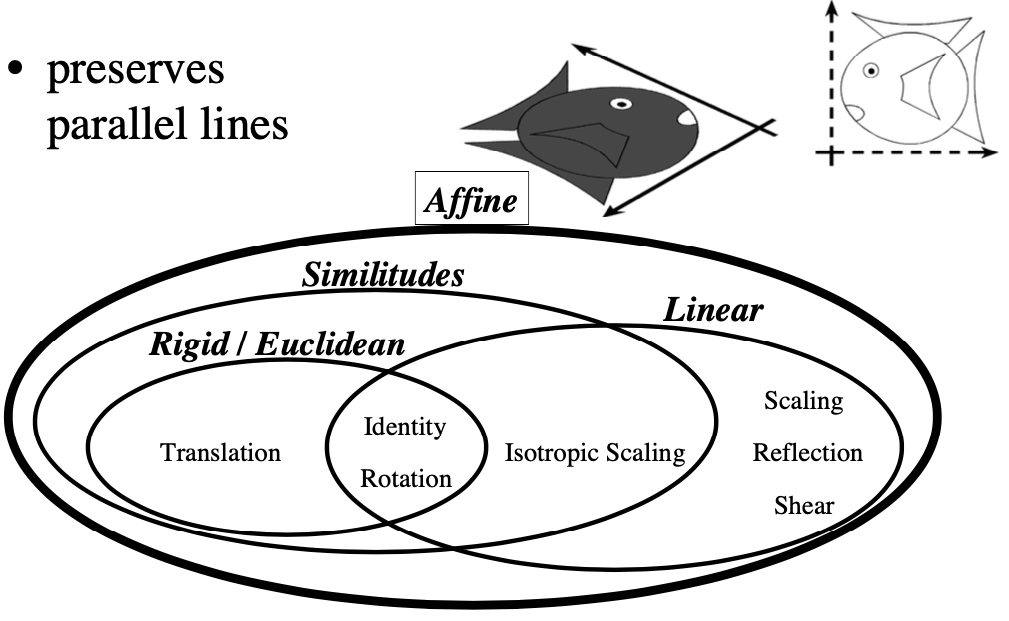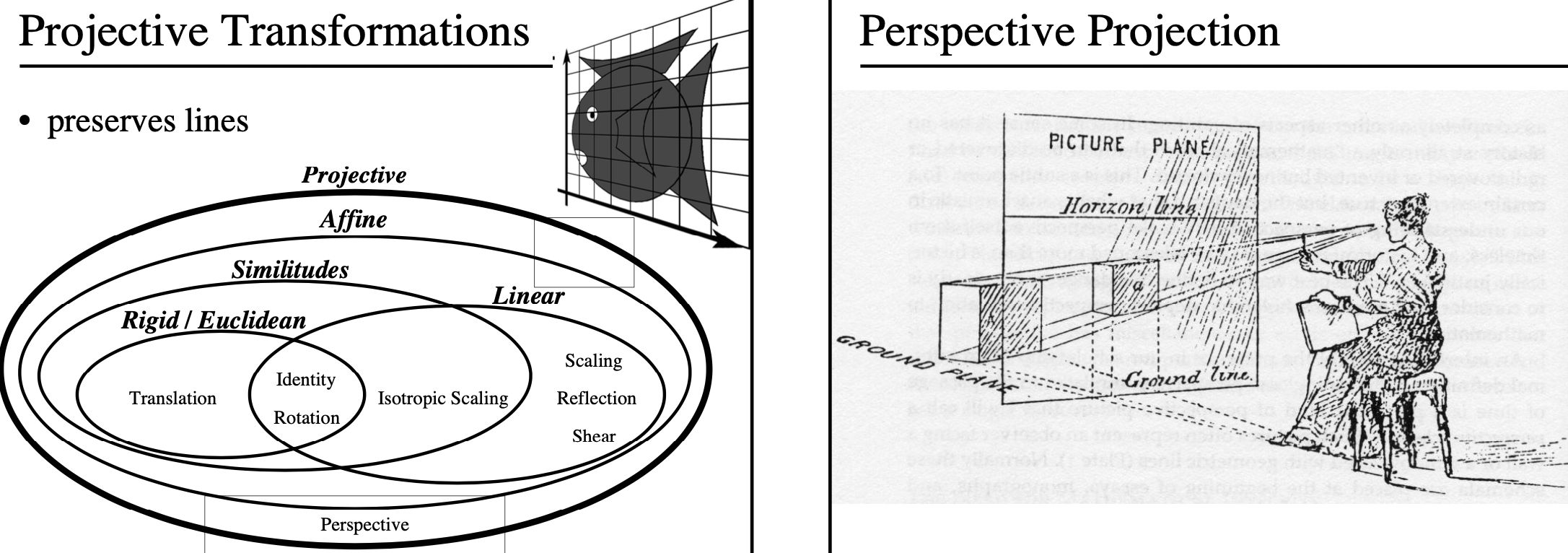## 仿射空间#

$$\mathbb{X}$$ 是向量空间，$$\pmb{L}\in\mathbb{X}$$ 是向量子空间，$$\pmb{a}\in\mathbb{X}$$ ，则 $$\pmb{a}+\pmb{L}=\{a+l:l\in\pmb{L}\}$$ 是仿射空间。这里的 $$\pmb{a}$$ 也称为平移向量。

## 仿射变换#

$\pmb{y}=\pmb{Ax}+\pmb{b}$

### 仿射变换的性质#

$$f(\pmb{x})=\pmb{Ax}+\pmb{b}$$ 是一个仿射变换，则 $$f$$ 具有：

1. 直线到直线的映射

2. 原来平行的直线变换之后仍然平行

1. 设直线 $$l:\pmb{p}+t\pmb{u},t\in\mathbb{R}$$ ，则：

$f(\pmb{p}+t\pmb{u})=\pmb{A}(\pmb{p}+t\pmb{u})+\pmb{b}=(\pmb{Ap}+\pmb{b})+t(\pmb{Au})=\pmb{p}_1+t\pmb{u}_1$

其中 $$\pmb{p}_1=\pmb{Ap}+\pmb{b}$$$$\pmb{u_1}=\pmb{Au}$$ ，则 $$f(l)=l_1, l_1:\pmb{p}_1+t\pmb{u}_1,t\in\mathbb{R}$$ 仍然是直线。

2. $$l:\pmb{p}+t\pmb{u}$$$$m:\pmb{q}+t\pmb{v}$$ 是平行线，则 $$\pmb{v}=k\pmb{u},k\in\mathbb{R}$$ ，所以：

$\begin{split} \begin{split} f(\pmb{p}+t\pmb{u})&=\pmb{A}(\pmb{p}+t\pmb{u})+\pmb{b}=(\pmb{Ap}+\pmb{b})+t(\pmb{Au})=\pmb{p}_1+t\pmb{u}_1 \\ f(\pmb{q}+t\pmb{v})&=f(\pmb{q}+t(k\pmb{u}))\\&=\pmb{A}(\pmb{q}+t(k\pmb{u}))+\pmb{b}\\&=(\pmb{Aq}+\pmb{b})+t(\pmb{A}k\pmb{u)}\\&=\pmb{q}_1+t(k\pmb{u}_1) \end{split} \end{split}$

故，变换之后所得 $$l_1:\pmb{p}_1+t\pmb{u}_1$$$$m_1:\pmb{q}_1+t(k\pmb{u}_1)$$ 仍然平行。

### 计算工具#

1. OpenCV

import cv2
import numpy as np
from matplotlib import pyplot as plt

rows, cols, ch = img.shape

pts1 = np.float32([[50, 50],
[200, 50],
[50, 200]])

pts2 = np.float32([[10, 100],
[200, 50],
[100, 250]])

# 构造对应点变换矩阵
M = cv2.getAffineTransform(pts1, pts2)
dst = cv2.warpAffine(img, M, (cols, rows))

plt.subplot(121)
plt.imshow(img)
plt.title('Input')

plt.subplot(122)
plt.imshow(dst)
plt.title('Output')

plt.show()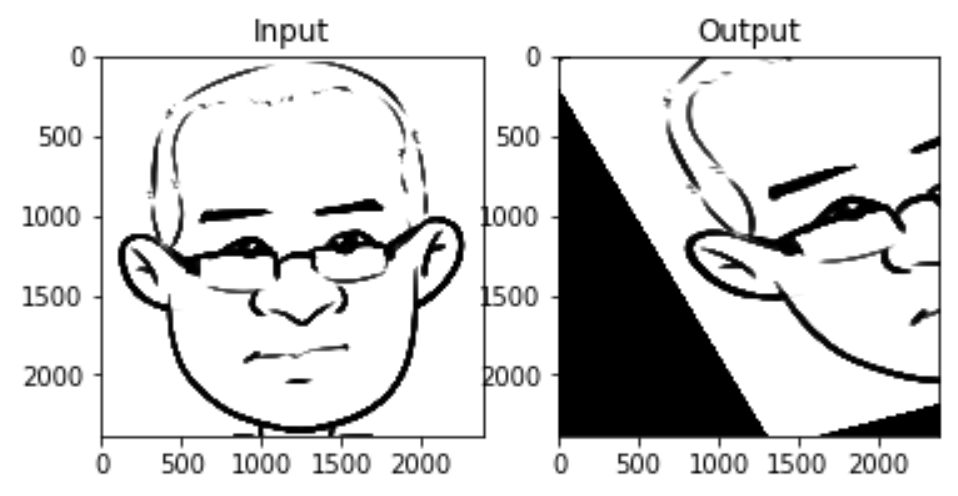2. 仿射变换模块

• Affine_transform：pip install affine-transform

• Affine：pip install affine，github仓库地址：https://github.com/sgillies/affine